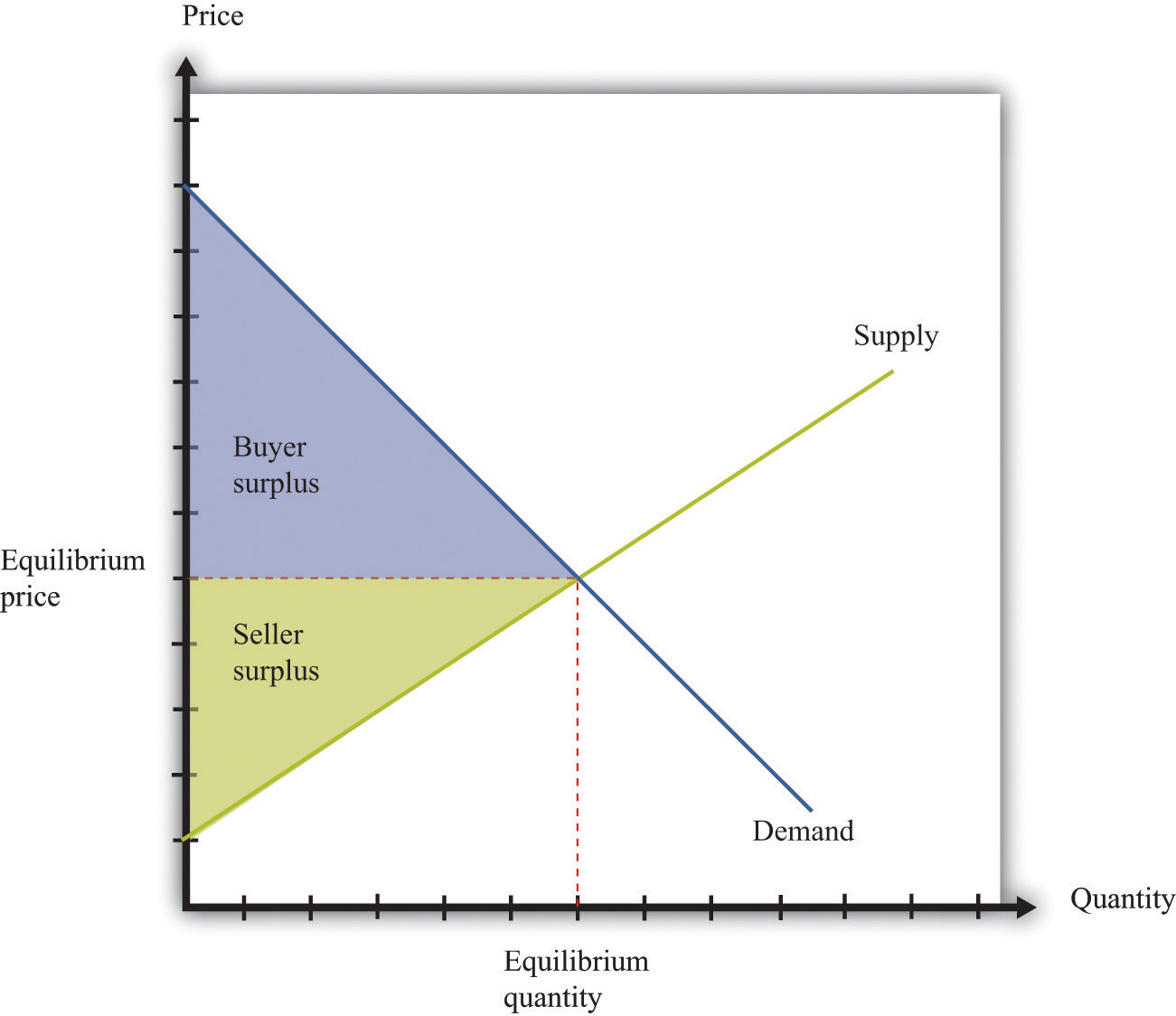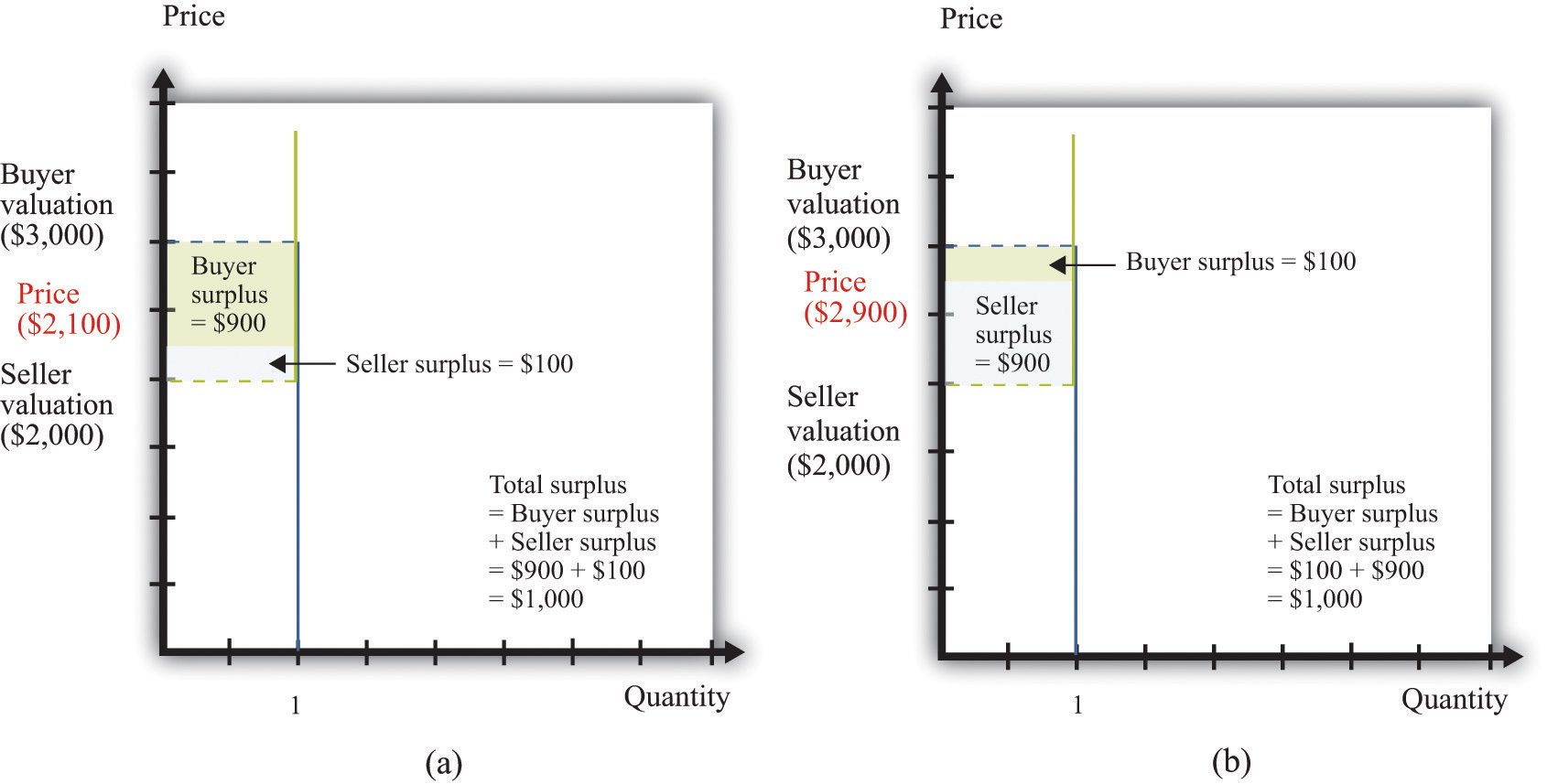## 17.10 Buyer Surplus and Seller Surplus

If you buy a good, then you obtain buyer surplus. If you did not expect to obtain any surplus, then you would not choose to buy the good.

• Suppose you buy a single unit of the good. Your surplus is the difference between your valuation of the good and the price you pay. This is a measure of how much you gain from the exchange.
• If you purchase many units of a good, then your surplus is the sum of the surplus you get from each unit. To calculate the surplus from each unit, you subtract the price paid from your marginal valuation of that unit.

If you sell a good, then you obtain seller surplus. If you did not expect to obtain any surplus, you would not sell the good.

• Suppose you sell a single unit of a good. Your surplus is equal to the difference between the price you receive from selling the good and your valuation of the good. This valuation may be a measure of how much you enjoy the good or what you think you could sell it for in some other market.
• If you sell many units of a good, then the surplus you receive is the sum of the surplus for each unit you sell. To calculate the surplus from selling each unit, you take the difference between the price you get for each unit sold and your marginal valuation of that extra unit.

Buyer surplus and seller surplus are created by trade in a competitive market (Figure 17.6 "A Competitive Market"). The equilibrium price and the equilibrium quantity are determined by the intersection of the supply and demand curves. The area below the demand curve and above the price is the buyer surplus; the area above the supply curve and below the price is the seller surplus. The sum of the buyer surplus and the seller surplus is called total surplus or the gains from trade.

Buyer surplus and seller surplus can also arise from individual bargaining (Figure 17.7 "Individual Bargaining"). When a single unit is traded (the case of unit demand and unit supply), the total surplus is the difference between the buyer’s valuation and the seller’s valuation. Bargaining determines how they share the gains from trade. The quantity of trades, indicated on the horizontal axis, is either zero or one. The valuations of the buyer and the seller are shown on the vertical axis. In this case, the valuation of the buyer (\$3,000) exceeds the valuation of the seller (\$2,000), indicating that there are gains from trade equal to \$1,000. How these gains are shared between the buyer and seller depends on the price they agree on. In part (a) of Figure 17.7 "Individual Bargaining", the buyer gets most of the surplus; in part (b) of Figure 17.7 "Individual Bargaining", the seller gets most of the surplus.

## Key Insights